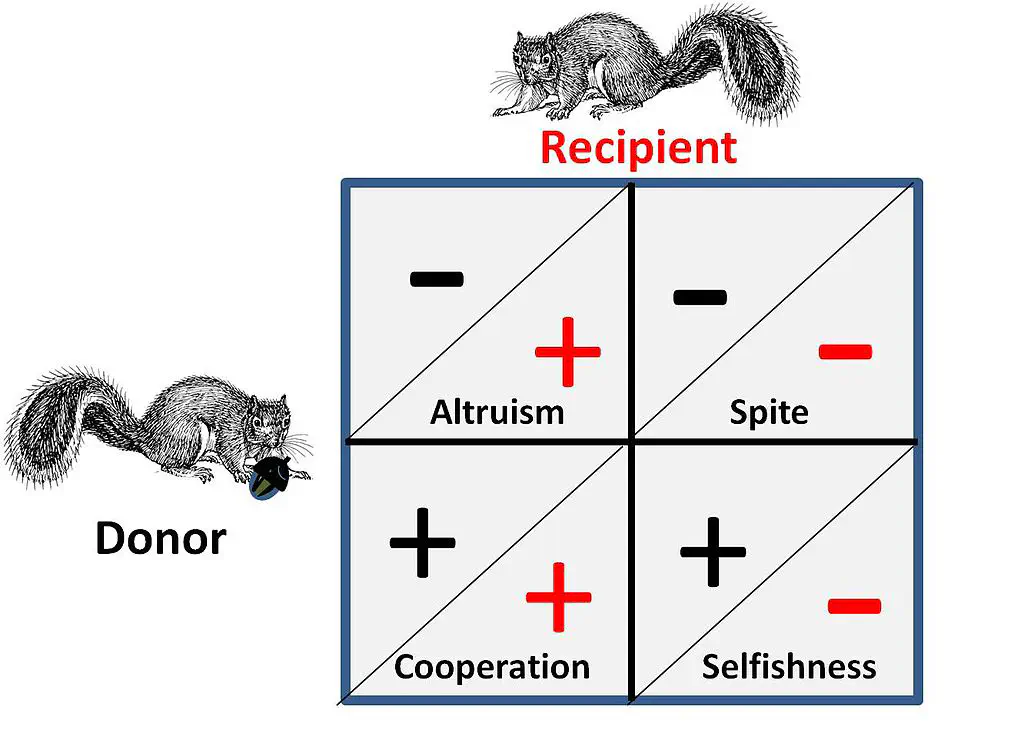# Fundamental mathematical models in evolution

This is a doctoral course aimed to provide a broad survey of well-known mathematical models in evolution.Image credit: from Wikipedia

## PhD course Fundamental mathematical models in evolution (Course coordinator)

This is a doctoral course aimed to provide a broad survey of well-known mathematical models in evolution. Emphasis is placed on understanding the basic formulation of each model, its underlying assumptions, its key results, and possible extensions. The course is inherently interdisciplinary and its intended audience is PhD students from mathematical and computational sciences as well as those from evolution and/or ecology. To facilitate student learning, after each lecture students were tasked with finding a relevant publication and giving a formal presentation on its motivation and use of the discussed mathematical model. Students would take turns presenting to one another in small groups and all presentations would be shared in a common directory.

• Lecture 1: Exponential growth
• Lecture 2: Price equation
• Lecture 3: Fitness landscapes
• Lecture 4: Hamilton’s rule
• Lecture 5: Evolutionary game theory
• Lecture 6: Trait groups
• Lecture 7: Models of neutral evolution
• Lecture 8: Evolutionary graph theory